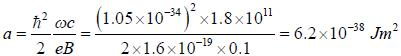Courses

# Test: Solid State Physics - 2

## 20 Questions MCQ Test GATE Physics Mock Test Series | Test: Solid State Physics - 2

Description
This mock test of Test: Solid State Physics - 2 for GATE helps you for every GATE entrance exam. This contains 20 Multiple Choice Questions for GATE Test: Solid State Physics - 2 (mcq) to study with solutions a complete question bank. The solved questions answers in this Test: Solid State Physics - 2 quiz give you a good mix of easy questions and tough questions. GATE students definitely take this Test: Solid State Physics - 2 exercise for a better result in the exam. You can find other Test: Solid State Physics - 2 extra questions, long questions & short questions for GATE on EduRev as well by searching above.
QUESTION: 1

### A monoatomic. cubic material has lattice spacing of a. The sound velocity for longitudinal and transverse phonons is approximately equal. CT = CL = C, is isotropic. The debye frequency of the phonons will be

Solution:

In debye model, the dispersion, related is approximated to be ω = ck and in 3-D case, the density of state is in the form of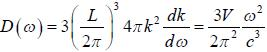where, V= L3 and the factor of 3 is due to the tact that there are one longitudinal and two transverse vibration modes for each k state.
If total number of states in solid is N. then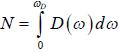Since, there are three states associated with each atom (2 for transverse and one for longitudinal vibration)
So,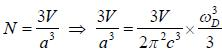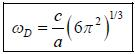QUESTION: 2

### Graphite lias a layered crystal structure in which the coupling between carbon atoms in different lasers is much weaker than the atoms in the same layer. Using Debye theory, the specific heat is proportional to (where T is the temperature)

Solution:

According to debye-model the density of states for a two dimension and lattice is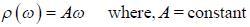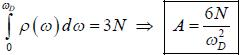Hence, total energy.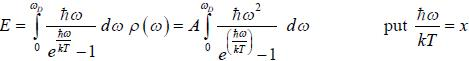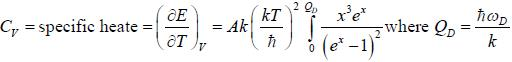For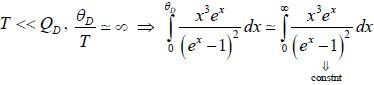⇒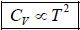QUESTION: 3

### All X-ray beam of wavelength 1.7 Å is diffracted by a cubic KC1 ciystal of density 1.99 x 103 kg m-3. The interplance spacing for (200) planes and the glancing angle for the second order reflection from these planes will be (given that molecular weight of KC1 is 74.6 amu)

Solution:

For cubic crystal we have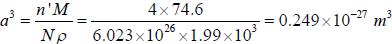n' = 4 because KC1 has fee structure
a = 6.29 x 10-10 m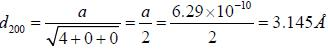From Bragg's law, 2d sin θ = nλ
n = 2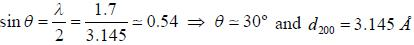QUESTION: 4

Elections of mass m are confined to one dimension. A weak periodic potential described by the fourier series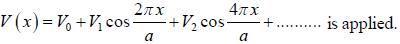The energy gap (to first order) at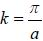(between the first and second band is)

Solution:

In such problems, the energy bands will be essentially parabolic with gaps at every point of degeneracy. This degeneracy is removed by mixing the corresponding wavefunctions and perturbation potential.
Solve the matrix element,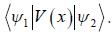Iii the neighbourhood of k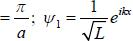(for lower band) and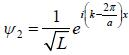or the upper band L is the length of sys te m ψ1 and ψ2 are calculated using extended zone
scheme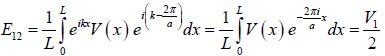W lien we diagnolise the Hamiltonia matrix we get the energy gap,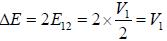QUESTION: 5

All electron of mass m moves in a square lattice of lattice spacing a. Assume that the nearly free electron approximation holds good. If there are two electrons per site, then which of the following is correct?

Solution:

With two electrons per site, assuming that the constant energy lines are circles, we obtain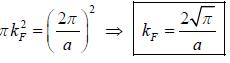71
This value is larger than π/a. Therefore, we needs to consider the two bands. There will be two fermi surface. a
one in the upper band and other in the lower band. Each of the band is partially filled, with two electrons per site the NFE approximation leads to a metal.

QUESTION: 6

A paramagnetic substance lias 1028 atoms/m3. The magnetic moment of each atom is 1.8x10-23 Am2. Wliat would be the dipole moment of a bar of this material 0.1 meter long and 1 cnr cross-section placed in a field of 8x 10Am-1?

Solution: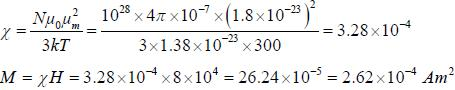QUESTION: 7

A uniform silver wire has a resistivity of 1.54 x 10-8 Clm at room temperature and there are 5.8 x 1028 conduction electrons per m3. If an electric field of 1 volt cm is applied along the wire, the relaxation time of electron will be

Solution:

Mobility,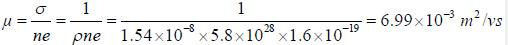Relaxation time τ is given by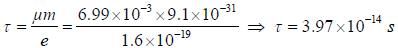QUESTION: 8

Assuming that there are 1027 molecules /m3 in HCl vapour. The oiientation polarization at room temperature if the vapour is subjected to an electric field of 106 V/m will be (given that dipole moment of HCl molecule is 3.46x10-30 c-m)

Solution:

Orientation polarizability is given by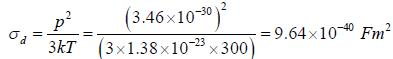Hence, the orientational polarization will given by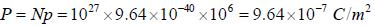*Answer can only contain numeric values
QUESTION: 9

Assume that the energy of the two particles in the field of each other is given by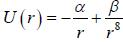where
α and β are cosntants and r is the distance between the centres of paiticles. In stable configuration, the ratio of energy of attraction to the energy of repulsion will be____________(Answer should be an integer)

Solution: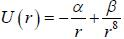Force,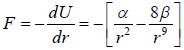For stable configuration, F= 0 at r - r0
⇒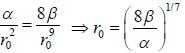E1=Energy of attraction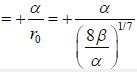E2 = Energy of repulsion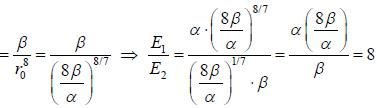*Answer can only contain numeric values
QUESTION: 10

Sodium transforms frombcc to hep at about T = 23 K. Assumig that the density remains fixed and c/a ratio is
ideal. The hcp lattice spacing, a [given that the cubic lattice spacing a' = 4.23Å in the cubic phase] is_______Å (Answer should be upto two decimal places)

Solution:

Density of bcc structure is 2 atoms per a'3 density of hep structue is 2 atoms per the volume of primitive unit cell V0.
Volume of unit cell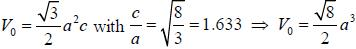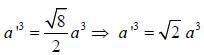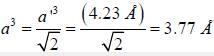QUESTION: 11

Atoms are arranged in a one-dimensional chain with lattice spacing a. Each atom is represented by the potential V(x) =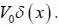. The energy gaps between the bands, assuming that the nearly free electron approximation holds good, is

Solution:

The energy gaps one determined by the fourier components of lattice potential. For the dirac-delta potentially. Tlie matrix elements are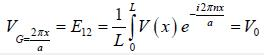Independent of n.
So, energy gap,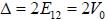QUESTION: 12

The effective mass tensor (Mij) for electrons in a simple cubic tight binding band at the centre (k = (0, 0,0) of the brillion zone will be

Solution:

The electron energy in tight binding approximation is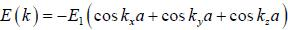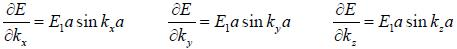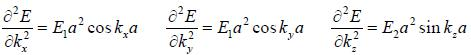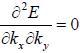Therefore, effective mass tensor,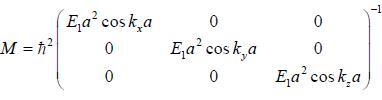At (0, 0, 0 )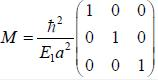QUESTION: 13

Consider a two dimensional hexagonal lattice if lattice spacing a = 3 Å and sound velocity c = 103 m/sec. The debye frequency, wD is (provide a numerical value in sec-1)

Solution:

The debye wave-number. kD is defined such that the total number of states within a radius of kD equals the total number of states in the Biillion zone.
⇒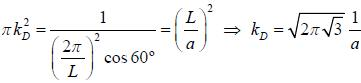⇒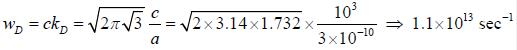QUESTION: 14

For a diatomic linear chain, the phonon dispersion relation ω (k) has two branches corresponding to + and - sign respectively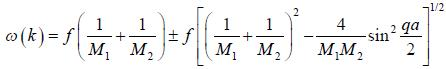There are two atoms in the unit cell with masses and M2 and the force constant of nearest neighbour interaction is F and the effective mass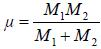is kept constant. The velocity of sound will be

Solution:

Sound velocity,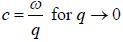Consider acquostic Branch, As q → 0, sin2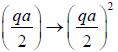⇒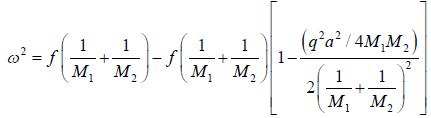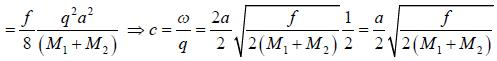QUESTION: 15

Consider a two dimensional square lattice with one atom of mass m per lattice point interacting with only nearest neighbours with force constant k. Take the phonon dispersion curve to be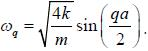At high temperature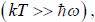the mean square displacement of an atom from its equilibrium position will be

Solution:

Total internal energy of the lattice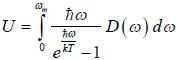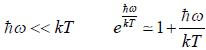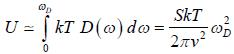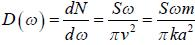If there are N atoms in lattice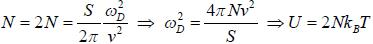At high temperature, average P.E. equals average K.E., hence half the total energy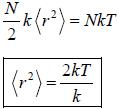QUESTION: 16

For a hexagonal closed packed structure with periodic potential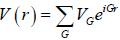(G being a reciprocal lattice G
vector). If G corrosponding to the first brillion zone face in the C direction C that is normal to the face is in the (001) direction), then the value of VG is

Solution: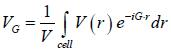If there are n identical atoms in the unit cell with positions. d1,d2........dj...,dn
Periodic potential.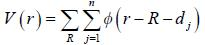where R is the position of lattice point.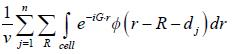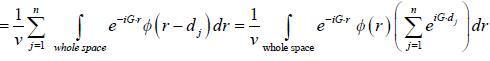Where,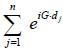is the geomatric structure factor
Unit cell of hcp contains 2 atoms at (0,0,0) and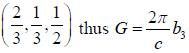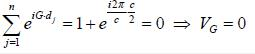QUESTION: 17

A type-II superconducting wire of radius R carries current uniformly through its cross-section. If die total current earned by the wire is I. the magnetic energy per unit length of the wire is I. the magnetic energy per unit length of the wire is

Solution: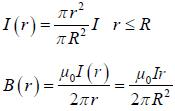Magnetic energy,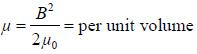Magnetic energy per unit length.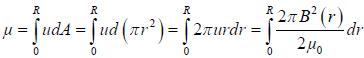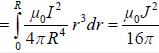QUESTION: 18

The penetrating depth of mercury at 3.5 k is about 750 Å. The penetration depth at OK will be

Solution:

From the formula for penetration depth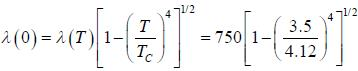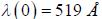*Answer can only contain numeric values
QUESTION: 19

Consider two dimensional electrons subjected to a weak periodic potential coming from a square lattice of spacing a = 5Å. For k vectors for away from the bu llion zone boundaries, the wavefunctions can be well
described by plane waves. Assume we want to write the wavefunction in block form,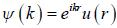. Consider a state of energy E and wavevector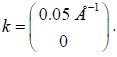The lowest energy at this wave number will be ________________eV (upto second decimal places)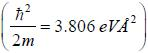Solution:

from schrodiner equation,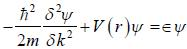⇒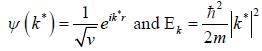where, k* extends over entire space.
So, we can transform k* into first B.z. by translation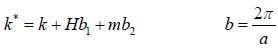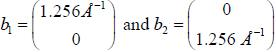For lowest energy,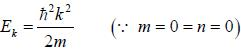= 3.806x 0.5 x 0.5 = 3.806x 0.25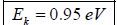*Answer can only contain numeric values
QUESTION: 20

Assume that the E vs k relationship for electrons in the conduction band of a hypothetical tetravalent n-type semiconductor can be approximated by E = ak2 + constant. The cyclotron resonane for electrons in a field B = 0.1 Weber/m2 occurs at an angular frequency ωe = 1.8 x 1011 rad s-1The value of a is_______x 10-38(in the untis of Jm2)

Solution:

Electron in conduction band behaves as if it had an effective mass m* given by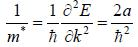Cyclotron resonance frequency for the electron is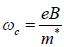⇒